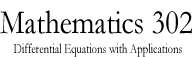Math 302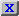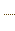HW3.pdfStatement on DisabilitiesStatement on EthicsHomework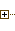Java Tools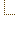Syllabus
 Math Dept. Home Help room

## 110.302 Differential Equations with Applications - Fall 2004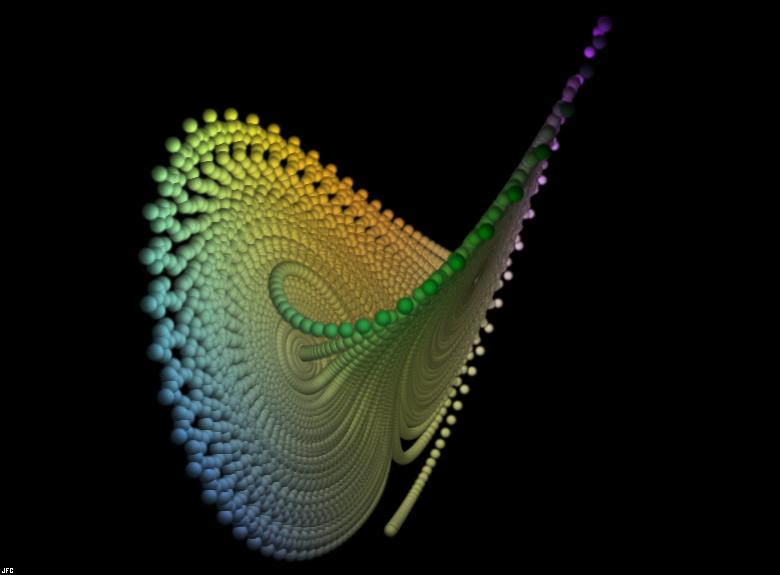This introductory course on differential equations emphasizes the techniques of solving ordinary differential equations, the properties of the solutions of these equations, and applications to engineering and the sciences. The topics to be covered include first order equations, trajectories, linear differential equations, power series solutions, Laplace transform, Fourier series, systems of linear differential equations, plane autonomous systems, critical points and stability. Applications to mechanics, heat conduction, vibrations, ecological systems and electric circuits will be discussed.